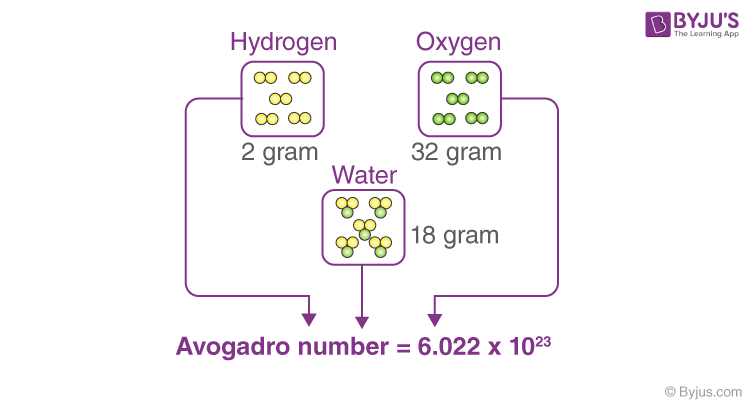Checkout JEE MAINS 2022 Question Paper Analysis : Checkout JEE MAINS 2022 Question Paper Analysis :

The measurement of large distances is simple. We use kilometers for terrestrial applications, light years for stellar applications, and 1 parsec (3.26 light-years) for galactic applications. On the other end of the scale, we have small measures. We can understand millimeters and our naked eyes can see up to 0.1 mm, but after that, it is very hard to visualize. We have only recently begun to explore the miniature world out there with the new technological development.An electron microscope is a microscope that illuminates the sample with a beam of accelerated electrons. This electron can be made to have a very short wavelength, almost 100,000 times shorter than the visible light, thus giving the electron microscope a better resolution than an optical microscope. This can be used to observe very small things, such as atoms and molecules. A transmission electron microscope can achieve better than 50 Picometers (10-12) resolution, and you should remember that atoms range from 30 to 300 Picometers. Here’s a fun fact, the radius of an atom is more than 10000 times the radius of the nucleus and the atom are 99.999999% empty space

Before the technological advancements though, we only had a rough estimate of the size of the atom even after the Rutherford alpha particle scattering experiment gave us the size of the nucleus. Let’s discuss some of these methods now.

The actual volume occupied by the atom of a substance is always less than the volume of that substance because the packing of atoms is inefficient. Due to this, there are empty spaces between atoms resulting in an inflated volume. According to Avogadro, the actual volume occupied by the atoms in a certain mass of a substance is two-thirds the volume occupied by that mass of the substance. Using the Avogadro Hypothesis and a few other parameters we can make an educated guess about the size of atoms.

Let’s take a mole of a substance, say carbon. A mole refers to the amount of the substance in grams equivalent to the atomic weight of the substance. So talking of carbon, one mole of carbon will contain 12 g of pure carbon-12 (12C). Fun fact: the definition of a mole as given by Avogadro uses carbon as the standard for mole.

We know that Volume is the ratio of mass by density. We can calculate the atomic volume of the whole mole.

Molar Volume = Molar Mass (gm)/ Density (gm/cm3)

A mole, by definition, has 6.02 x 1023 elementary particles. Using this we can obtain the atomic volume of the carbon atom.

Atomic volume = Molar Mass (gm)/ [Density (gm/cm3) x Avogadro Number]

Carbon Atomic Volume = 12 gm/ [(2 gm/cm3) x) 6.02 x 1023)]

We must also remember to introduce the two-thirds law by Avogadro.

Carbon Atomic Volume = [2 x 12 gm]/ [3 x (2 gm/cm3) x) 6.02 x 1023)] = 6.6467 x 10-24 cm3

Calculating the diameter, we get a value of

Carbon Diameter = 0.116 nm

This is not correct. This value is a little too small. The typical atom diameter starts from 0.3 nm i.e. 30 pm and yet this was the first considerably accurate estimate ever made. This is what made the Avogadro hypothesis exciting.

## Frequently Asked Questions – FAQs

Avogadro’s hypothesis states that the total number of atoms/molecules of a gas is directly proportional to the volume occupied by the gas at constant temperature and pressure.

### What is an ideal gas?

An ideal gas is a theoretical gas composed of a set of randomly-moving point particles that interact only through elastic collisions.

PV = nRT

### What is meant by gas constant?

It is the physical constant expressed in terms of units of energy per temperature increment per mole.

### What is gas constant also known as?

Ideal gas constant Ideal gas constant or molar gas constant or universal gas constant.